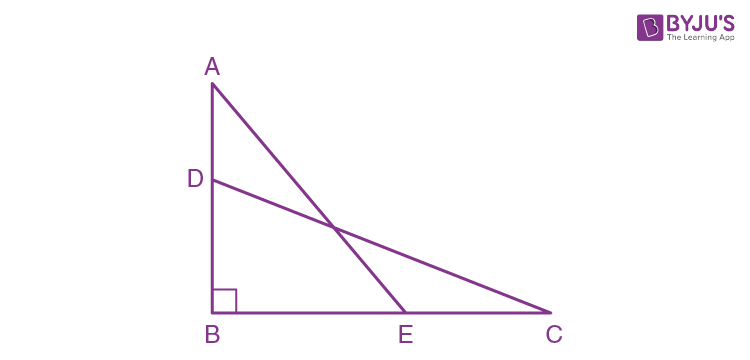# In the adjoining figure, AE = DC = 13 cm BE = 5 cm, ∠ABC=90°& AD = EC = x cm. Calculate the length of AB & the value of x

Now, consider a triangle ABE,By using Pythagoras theorem,

AE2 = AB2 + BE2

169 = AB2 + 25

AB2 = 169 – 25 = 144.

Hence, AB = 12.

Now, consider the triangle DBC, and use pythagoras theorem,

DC2 = DB2 + BC2

169 = (AB – x)2 + (5+x)2

169 = AB2 + x2 – 2xAB + 25 + x2 + 10x

169 = 144 + x2 – 24x + 25 + x2 + 10x

169 – 144 = x2 – 24x + 25 + x2 + 10x

25 = x2 – 24x + 25 + x2 + 10x

2x2 – 14x + 25 – 25 = 0

2x2 – 14x = 0

2x (x – 7) = 0

⇒2x = 0, x = 0

⇒ (x-7) = 0

⇒ x = 7.

Hence, the value of x is 7.(1)(0)